# New Method of Determining the Time and the Pole Height from the Height of Two Stars, together with Astronomical Observations, by Professor Gauss in Göttingen. 1)

### Translated from the Latin by Prof. Harding there, and sent in under May 29, 1809.

1.

As is well known, travelling astronomers usually make use of observations of the sun to determine geographical positions, and to determine the time from its corresponding altitudes, as well as the altitude of the poles from their altitudes in or near the meridian. In fact, these observations are recommended from several sides, especially if one uses a mirror sextant to measure the altitude, since with it the altitudes of the sun can be measured much more precisely than those of the stars, and the reading of the limbus is easier by day than by night; also, the calculation of the altitudes of the sun in and near the meridian is so simple that it cannot be difficult even for those who have little mathematical knowledge. Even if unfavourable weather does not allow us to obtain the corresponding altitudes of the sun, the status of the clock can be easily derived by combining the altitudes far from midday, and also the polar altitude by indirect methods, e.g. Douwes‘.

With all these decisive advantages of ⊙observations, one would be wrong if one wanted to neglect the stellar altitudes altogether. Very often the sky brightens up at nightfall, or at least allows star elevations to be measured for a short time; often time does not allow the traveller to stay during the day, and he would therefore be able to make a contribution to geography if he had the necessary skill to determine the position of the place where he is staying overnight from star observations. If one adds to this the uncertainty of the observations that the low position of the sun makes unavoidable in winter, as well as the inconvenience that the observer experiences from the crowding of curious onlookers or from the shaking of his artificial horizon by passing carriages, all of which is largely absent during the still night, then one will always have to concede a not insignificant value to star observations.

These observations are especially recommended to seafarers, for whom it is of the utmost importance for the preservation of their ship and often their very lives to determine the location of the ship every day if possible. It is true that on the sea it is always difficult to recognise the true horizon with the necessary accuracy in the dark of night: but a telescope with a large aperture, which can be attached to the sextant, will easily overcome this obstacle.

2.

Another very considerable advantage of this method is that within a few minutes both the altitude of the pole and the time can be determined with immense precision. For this, nothing more is required than to measure the altitude of two stars whose position is known, and to note the time of the measurements indicated by the clock: the daily course of the clock need only be known to such an extent that from it the intermediate time of the observations can be converted with accuracy into sidereal time.

This problem is undoubtedly one of the most useful in nautical astronomy, and it is therefore surprising that not a single one of the writers who have presented this science discusses it. It is true that Mr. Kraft (Act. nov. Acad. Petrop. T. XIII.) teaches us to determine the Pole height (altitude) from the altitudes of two stars; but, strangely enough, he restricts this general problem by the special condition that both altitudes should be measured at one and the same moment. This necessarily requires two observers, two measuring instruments and at the same time the most exact agreement in the observations, preconditions that cannot be met so easily. Moreover, this condition is also completely superfluous, since every well-equipped ship will certainly be equipped with a clock, the movement of which can be relied upon within a few minutes, the ship does not noticeably change its geographical position in such a short time, and finally, the whole task is not simplified by this condition in any way with regard to the calculation.

3.

It can easily be shown that the problem, as Mr. Kraft has treated it, can also be expressed thus: „From the given position of two points on the surface of the sphere in relation to a largest circle (which here is the equator), find the position of a third point, whose distances from these points are given“. The first two points are given by the position of the two stars observed on the celestial sphere, whose straight ascension and deviation are assumed to be known; the third point is the zenith of the place of observation, whose declination is equal to the pole height, and whose straight ascension is the culminating point of the equator, and consequently also determines the sidereal time. Accordingly, this task is based on the resolution of three spherical triangles; the first lies between the two given points and the pole of the equator, in which two sides are given together with the included angle, and through which the third side and one of the two remaining angles are found. The second triangle includes these two given points and the point sought: in this all three sides are already known, and an angle is derived from it, either that at the first, or that at the second point, depending on whether in the first triangle one had chosen that at the first, or that at the second point, as the angle sought. The sum or difference of these two angles determines an angle in the third triangle, which the first and third points and the pole of the equator enclose, and in which two sides are known in addition to that angle. From this, the angle at the pole and the distance of the third point from the pole (which is equal to the complement of the pole height) can be found.

Already in the 16th century, astronomers made use of this task, see, among others, Tychoni’s Astron. instaur. progym. P. 221 etc. where the position of many stars is determined from the distance between two known stars.

At the same time, it is easy to see from the above representation that this problem allows two solutions each time: for if one draws two circles from two given points of the spherical surface with the radii, which are equal to the given distances between them, then these will intersect each time at two points, in each of which the point sought for can lie. From this, however, no ambiguity can arise in the application: for if one imagines a largest circle laid through the first and second point, which thus divides the surface of the sphere into two hemispheres, there can be no further doubt whether the third point (the zenith) and the north pole lie in the same hemisphere or in different ones, and it is clear that the first case must take place, if the excess of the straight ascent of the star observed to the left of the other over the straight ascent of the other star (after the first AR increased by 360° if necessary) falls between 0° and 180°, whereas the second case occurs if the same excess falls between 180° and 360°.
Incidentally, it is precisely this ambiguity of the problem, which lies in the nature of the matter, that is to be regarded as the cause of the somewhat extensive calculations to which the direct solution of the problem leads: however, it will be shown below how this can be noticeably shortened. If, however, one prefers an indirect resolution, this can be made immensely supple, as I shall show on another occasion.
What has been said so far refers to the special case when both observations are simultaneous; in general, however, it can be represented as follows: instead of the second observed star, imagine a point on the celestial sphere which has the same deviation as this second star, but an AR so much smaller than the sidereal time which has elapsed between the two observations. It is clear that this imaginary star would have reached the same height at the time of the first observation as the star actually observed had at the moment of the second observation. From this it follows that if one takes the imaginary star for the real one, the calculation is based on the case of the simultaneous observation.

All this is based on purely geometrical, admittedly quite simple considerations; but it will doubtless be agreeable to many to see a direct solution of this problem developed in a purely analytical way, which will confirm anew that all truths which are derived from geometrical considerations can be discovered just as elegantly with the aid of analysis, if it is only treated in the right way.

4.

Letbe the polar altitude, α and α‘ the straight ascension, δ and δ‘ the declination of the two stars, γ and γ‘ the straight ascension of the culminating points of the equator at the moment of the first and second observation, or whichever is the case, the sidereal times themselves transformed into degrees; h the observed altitude of the first, h‘ that of the second star 2), from which it follows that γ – α and γ‘ – α‘ are the hour angles associated with the two observations. Let γ – α =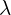; γ‘ – α‘ =‚ – θ, from which θ = α‘ – α – (γ‘ – γ) and consequently is known because γ‘ – γ is the sidereal time transformed into degrees between the two observations.
Accordingly, the solution of this problem is based on the development of the following two equations:

(1)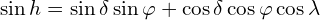(2)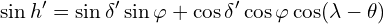The elimination of one of the two unknown quantitiesorwould lead to a very complicated equation. Mr. Kraft has chosen this path in his dissertation, but its resolution is, in my opinion, incomparably more extensive and laborious than the one that results directly from the consideration of three triangles, although it is restricted only to the determination ofand does not get involved in the determination of time. It is therefore better to eliminate bothandwith the help of a new unknown quantity: the following remark shows the way to its appropriate choice. It is obviously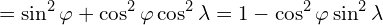and therefore according to equation (1)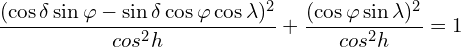One can therefore set

(3)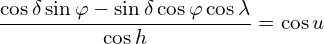(4)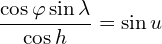If equations 3 and 1 are now connected, the following follows

(5)(6)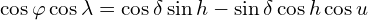Let equation 2 take the following form: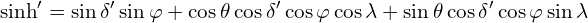and set for sin φ; cos φ ⋅ cos; cos φ ⋅ sin; their values of 5, 6 and 4, then we find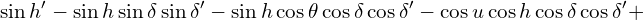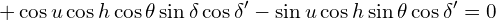This equation transforms if one sets

(7)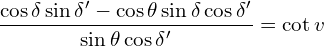in the following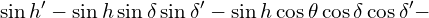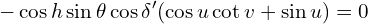If one further makes v – u = w , then one has

(8)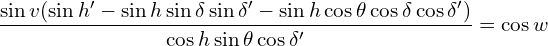from which therefore w and also u = v – w become known.After the angle u has been found,can now also be easily derived from the connection of equations 4 and 6, which results in

(9)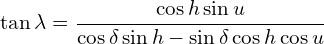But ifis known, then γ = α +also results, and if one transforms this angle into time, then one has the status of the clock. From the connection of equations 4 and 5 we get

(10)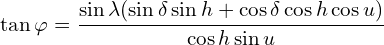Equation 4 can be connected to this formula, so that the correctness of the calculation can be checked at the same time.

5.

Concerning the resolution taught in the preceding part, I note the following: since the angle w is found by the cosine according to formula 8, two values are possible for it, a positive and a negative one, and the task therefore permits a double resolution, as has already been noted above: but it can be easily decided in the following way which of the two values is the true one in each case that occurs. An easy observation shows, then, that the sine of the excess of the azimuth of the first star over the azimuth of the second star, counted from the left to the right hand, will be (sin θ cos δ‘ sin w)/(cos h‘ sin v). From this it follows immediately that, since cos δ‘ and cos h‘ are by their nature positive quantities, sin w/sin v must also have the same or opposite sign, depending on whether the vertical circle passing through the first star lies on the right or left hand from that passing through the second star. But there can never be any doubt about this case, since such stars whose vertical circles either fall close together or have almost an opposite position are not useful for these observations and must therefore be carefully avoided, as will be explained in more detail below.

Incidentally, the resolution given above can also be shortened somewhat and made more convenient for numerical development by introducing two auxiliary angles. If one then sets

(11)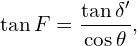so equations 7 and 8 transform into the following:

(12)(13)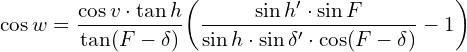Furthermore, call a second auxiliary angle G, and make

(14)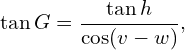equations 9 and 10 transform into the following:

(15)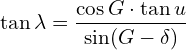(16)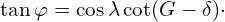6.

Practical astronomers not infrequently complain that the methods proposed by the theorists are not always entirely suitable for real application, or at least do not fully achieve what they expect of them. That this reproach does not apply to the method presented here will be adequately demonstrated by the following example, for which I borrow the data from actual observations. With a Troughtonian sex constant of 10 inches, I found the double and improved height of α in the eagle on August 21, 1808 …

1) Extract from the publication by C. F. Gauss, published in “Astronomisches Jahrbuch für das Jahr 1812“, Berlin 1809

2) In the monthly Correspondence of February 1809, where there is a short extract from this treatise, this problem is presented as if it required the same altitudes of the stars. That this is not at all the case, however, is already evident from the different designation that the author has given to the two heights. Incidentally, this method would also lose much of its convenience and usefulness through such a requirement. H.